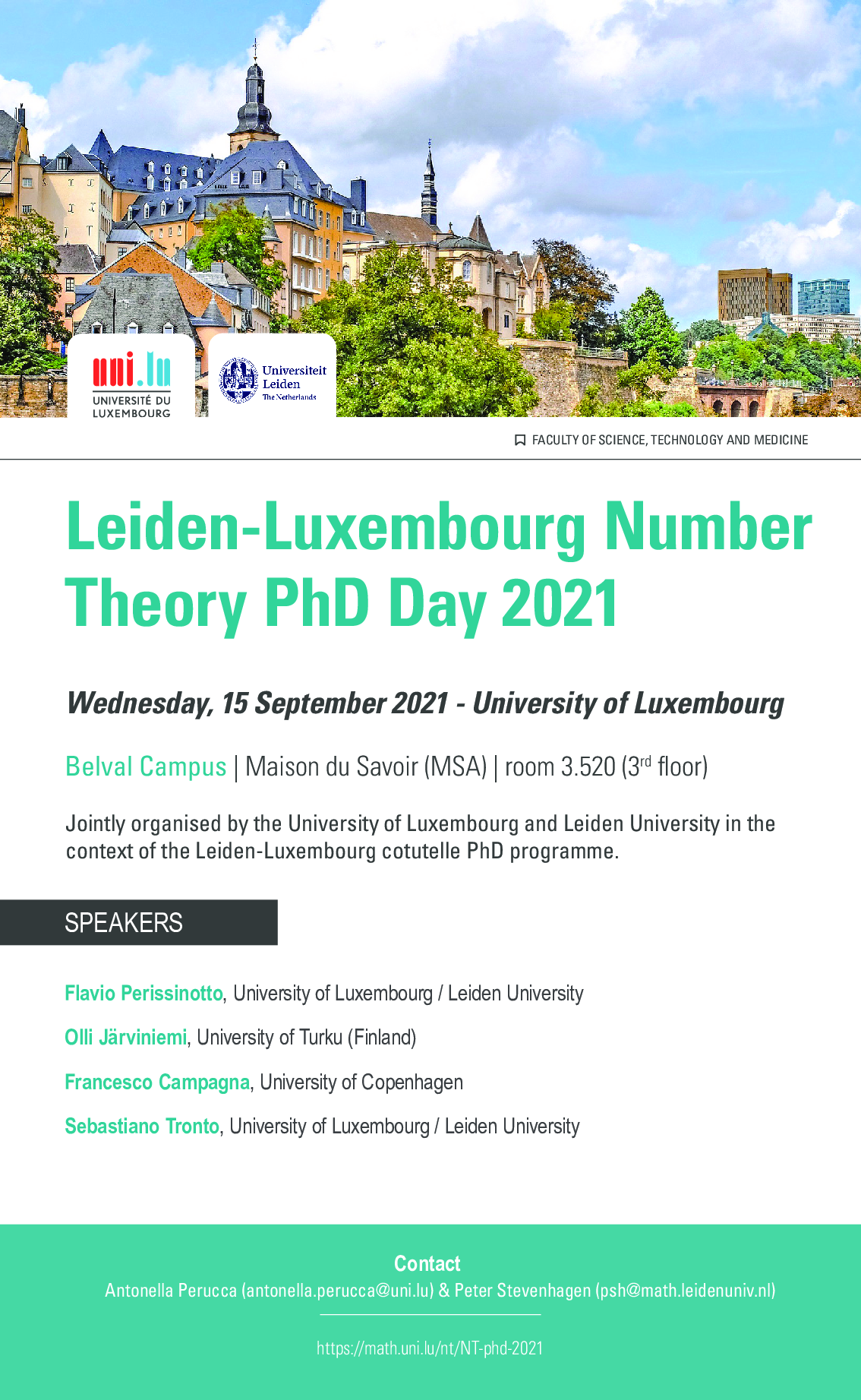# Leiden-Luxembourg Number Theory PhD Day 2021

### September 15, 2021Location: University of Luxembourg, Campus Belval, Maison du Savoir, room 3.520

Webex link: Available on request, contact antonella.perucca@uni.lu

 Time Speaker Title 12:10-12:50 Flavio Perissinotto (University of Luxembourg / Leiden University, the Netherlands) Kummer theory for multiquadratic and quartic cyclic number fields 12:50-14:00 Lunch Break 14:00-14:40 Olli Järviniemi (University of Turku, Finland) Higher degree Artin conjecture 15:00-15:40 Francesco Campagna (University of Copenhagen, Denmark) Reduction of elliptic curves and cyclic groups 16:00-16:40 Sebastiano Tronto (University of Luxembourg / Leiden University, the Netherlands) Division in modules and Kummer Theory

#### Abstracts

Flavio Perissinotto Kummer theory for multiquadratic and quartic cyclic number fields

Let K be a multiquadratic or quartic cyclic number field and let G be a finitely generated subgroup of its multiplicative group. In this talk we show that there is a finite procedure to compute at once, for all integers M and N with N dividing M, the degree of the cyclotomic-Kummer extension of K realized by adding the M-th roots of unity and the N-th roots of the elements in G over the cyclotomic extension of K. We also present a direct application of the result on the analogous problem related to torsion-Kummer extensions for a finite product of 1-dimensional tori defined over Q. (Joint work with Antonella Perucca)

Olli Järviniemi Higher degree Artin conjecture

We consider a generalization of Artin's primitive root conjecture to algebraic numbers: given an algebraic number, what can we say about the multiplicative orders of its reductions to finite fields of varying characteristic? The problem becomes increasingly difficult when the degree of the number or its reduction are increased. We survey the status of the conjecture and present some new results.

Francesco Campagna Reduction of elliptic curves and cyclic groups

Let E be an elliptic curve defined over a number field K. A prime of cyclic reduction for E is a prime of K modulo which E has good reduction and such that the point group on the reduced curve can be generated by a single element. In this talk we explain why the set S=S(E/K) of primes of cyclic reduction for E should have a natural density and we discuss the causes that make this density equal to zero. The case of E having complex multiplication will be of particular interest. This is joint work with Peter Stevenhagen.

Sebastiano Tronto Division in modules and Kummer Theory

If G is a commutative algebraic group over a number field K, one can consider the set of points of G over an algebraic closure of K that are multiples of K-points. The extension of K generated by the coordinates of these points is in many way an analogue of the Kummer extensions, i.e. extensions generated by the n-th roots of some fixed elements of K. Many properties of this field extension can be studied using a certain exact sequence of Automorphism groups. For example, if G is an elliptic curve without complex multiplication then from Serre's Open Image Theorem one can derive an open image theorem for this type of Kummer extensions. On the other hand, if the elliptic curve does have complex multiplication, it turns out that one needs to consider these groups of points as End(G)-modules, as illustrated by Abtien Javan Peykar in his recent PhD thesis. In this talk outline a recent attempt (work in progress) of generalizing these results.

Jointly organized by the University of Luxembourg and Leiden University in the context of the Leiden-Luxembourg cotutelle PhD programme.

Contact: Antonella Perucca, Peter Stevenhagen